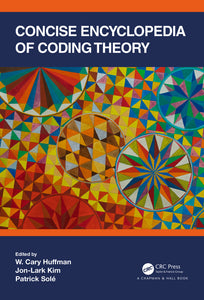# Concise Encyclopedia of Coding Theory

Regular price \$250.00 \$250.00 Sale

Author(s):

Most coding theory experts date the origin of the subject with the 1948 publication of A Mathematical Theory of Communication by Claude Shannon. Since then, coding theory has grown into a discipline with many practical applications (antennas, networks, memories), requiring various mathematical techniques, from commutative algebra, to semi-definite programming, to algebraic geometry.

Most topics covered in the Concise Encyclopedia of Coding Theory are presented in short sections at an introductory level and progress from basic to advanced level, with definitions, examples, and many references.

The book is divided into three parts:

Part I fundamentals: cyclic codes, skew cyclic codes, quasi-cyclic codes, self-dual codes, codes and designs, codes over rings, convolutional codes, performance bounds

Part II families: AG codes, group algebra codes, few-weight codes, Boolean function codes, codes over graphs

Part III applications: alternative metrics, algorithmic techniques, interpolation decoding, pseudo-random sequences, lattices, quantum coding, space-time codes, network coding, distributed storage, secret-sharing, and code-based-cryptography.

Features

• Suitable for students and researchers in a wide range of mathematical disciplines
• Contains many examples and references
• Most topics take the reader to the frontiers of research

Review(s):

"If you want a comprehensive outline of classical coding theory and its modern applications, you will appreciate this encyclopedia. It is written by a large international group of experts with a wide spread of experience. Extensive references to publications and web sources support the chapters; they allow the reader to follow in depth interests sparked by each topic covered. The vast range of applications detailed makes it evident that coding theory is a major and vital area of mathematics."
– Emeritus Professor Harold Ward, University of Virginia

EAN: 9781351375108